Courses
Courses for Kids
Free study material
Offline Centres
MoreLast updated date: 28th Nov 2023
Total views: 279.6k
Views today: 7.79k

# Construct the angles of the following measurements:1. $30^\circ$ 2. $22\dfrac{{1^\circ }}{2}$ 3. $15^\circ$Verified
279.6k+ views
Hint: We have to construct angles of measurement $30^\circ ,22\dfrac{{1^\circ }}{2}$ and $15^\circ$ . For that, first of all draw a horizontal line segment of any length you want. Name the end points of this line segment A and B. Now, place the midpoint of the protractor at point A and mark a point at $30^\circ ,22\dfrac{{1^\circ }}{2}$ and $15^\circ$ respectively. Now, join the point with vertex A and we will get our angle.

In this question, we have to construct an angle measuring $30^\circ ,22\dfrac{{1^\circ }}{2}$ and $15^\circ$ respectively. We will be using the protractor for constructing these angles.
Steps for constructing an angle using protractor:

1) $30^\circ$
Step 1:
First of all, we need to construct a base. So, draw a horizontal line segment of any measure you wish.
Name these endpoints of the line segment A and B.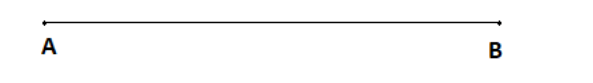Step 2:
Now, we have to construct an angle $\angle A = 30^\circ$ .
For that, place the midpoint of the protractor at point A and then mark a point at angle 30.
Now, join that point to the vertex A.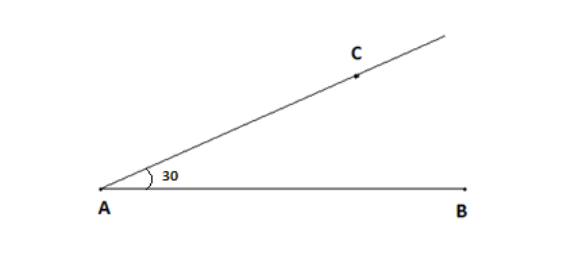Hence, we have constructed an angle $\angle CAB = 30^\circ$ .

2) $22\dfrac{{1^\circ }}{2}$
Step 1:
First of all, we need to construct a base. So, draw a horizontal line segment of any measure you wish.
Name these endpoints of the line segment A and B.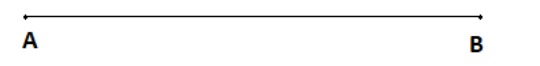Step 2:
Now, we have to construct an angle $\angle A = 22\dfrac{{1^\circ }}{2}$ .
For that, place the midpoint of the protractor at point A and then mark a point at angle $22\dfrac{1}{2}$ .
Now, join that point to the vertex A.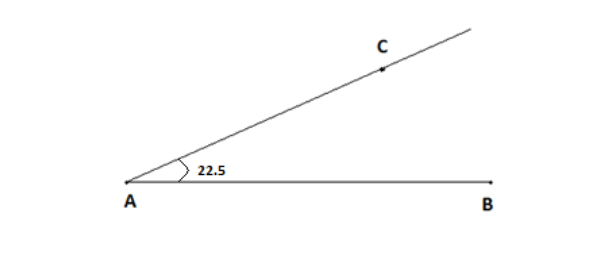Hence, we have constructed an angle $\angle CAB = 22\dfrac{{1^\circ }}{2}$ .

3) $15^\circ$
Step 1:
First of all, we need to construct a base. So, draw a horizontal line segment of any measure you wish.
Name these endpoints of the line segment A and B.Step 2:
Now, we have to construct an angle $\angle A = 15^\circ$ .
For that, place the midpoint of the protractor at point A and then mark a point at angle 15.
Now, join that point to the vertex A.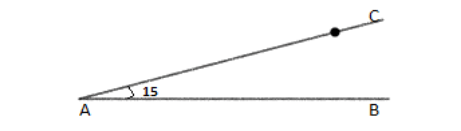Hence, we have constructed an angle $\angle CAB = 15^\circ$ .

Note: Angles can also be constructed using ruler and compass. For that you need to draw a copy of the angle you are constructing and then draw an arc of any radius on that copy. Then, you have to draw another line segment of any measure and draw an arc of the same radius used before. Now, you need to measure the length of the arc drawn in the copy and use that length to mark a point on the other arc we have drawn. Now, simply draw the line segment passing from that point and the vertex.